# A spring oscillator is designed with a mass of 0.193 kg. It operates while immersed in...

A spring oscillator is designed with a mass of 0.193 kg. It operates while immersed in a damping fluid, selected so that the oscillation amplitude will decrease to 1.00% of its initial value in 9.35 s. Find the required damping constant for the system.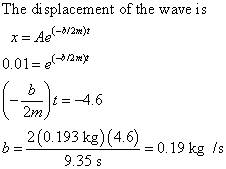#### Earn Coin

Coins can be redeemed for fabulous gifts.

Similar Homework Help Questions
• ### A horizontal spring of spring constant k = 125N/m is resting at its equilibrium length. One end o...

A horizontal spring of spring constant k = 125N/m is resting at its equilibrium length. One end of the spring is attached to a wall, and the other end is attached to a mass M = 200g. The whole system is immersed in a viscous fluid with linear drag coefficient b = 0.1 kg/s. The mass is pulled 10cm from its equilibrium position and released from rest. (a) (0.5 pt) What would be the oscillation period if the system were...

• ### A mass of 1.32 kg is connected to a spring of spring constant 8.81 N/m ....

A mass of 1.32 kg is connected to a spring of spring constant 8.81 N/m . An oscillation is started by pulling the mass to the right to amplitude 0.582m before release and the oscillator moves in air. The oscillation decays to 18.2% of the original amplitude in 58.2 seconds. the damping constant of the oscillation is 7.73*10^-2 kg/s total energy has the system lost in this time due to air damping = 1.44 j the amplitude of the oscillation...

• ### A 9.10 kg object oscillates at the end of a vertical spring that has a spring...A 9.10 kg object oscillates at the end of a vertical spring that has a spring constant of 2.25 times 10^4 N/m. The effect of air resistance is represented by the damping coefficient b = 3.00 N s/m. Calculate the frequency of the dampened oscillation. By what percentage does the amplitude of the oscillation decrease in each cycle? Find the time interval that elapses while the energy of the system drops to 3.00% of its initial value.

• ### A car and its suspension system act as a block of mass m= on a vertical spring with k 1.2 x 10 N m, which is damped...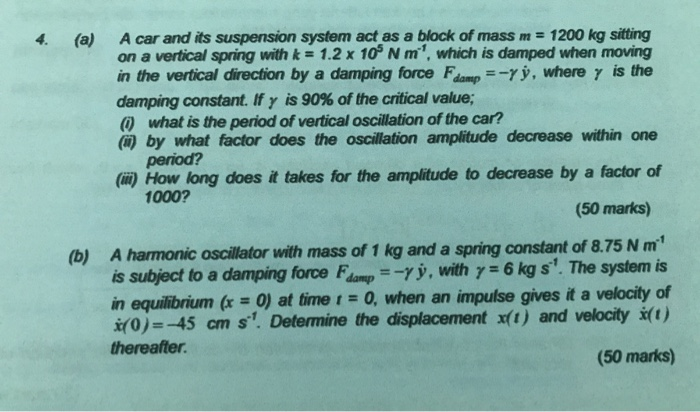A car and its suspension system act as a block of mass m= on a vertical spring with k 1.2 x 10 N m, which is damped when moving in the vertical direction by a damping force Famp =-rý, where y is the 1200 kg sitting 4. (a) damping constant. If y is 90% of the critical value; what is the period of vertical oscillation of the car? () by what factor does the oscillation amplitude decrease within one period?...

• ### An undamped 2.19 kg horizontal spring oscillator has a spring constant of 34.5 Nm. While oscillating,...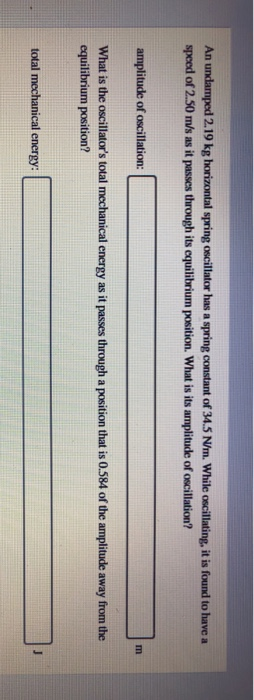An undamped 2.19 kg horizontal spring oscillator has a spring constant of 34.5 Nm. While oscillating, it is found to have a speed of 2.50 m/s as it passes through its equilibrium position. What is its amplitude of oscillation? amplitude of oscillation: What is the oscillator's total mechanical energy as it passes through a position that is 0.584 of the amplitude away from the equilibrium position? total mechanical energy:

• ### An undamped 1.02-kg horizontal spring oscillator has a spring constant of 29.9 N/m. While oscillating, it...

An undamped 1.02-kg horizontal spring oscillator has a spring constant of 29.9 N/m. While oscillating, it is found to have a speed of 2.21 m/s as it passes through its equilibrium position. What is its amplitude of oscillation? What is the oscillator\'s total mechanical energy as it passes through a position that is 0.701 of the amplitude away from the equilibrium position?

• ### An undamped 2.87 kg horizontal spring oscillator has a spring constant of 26.5 N/m. While oscillating,...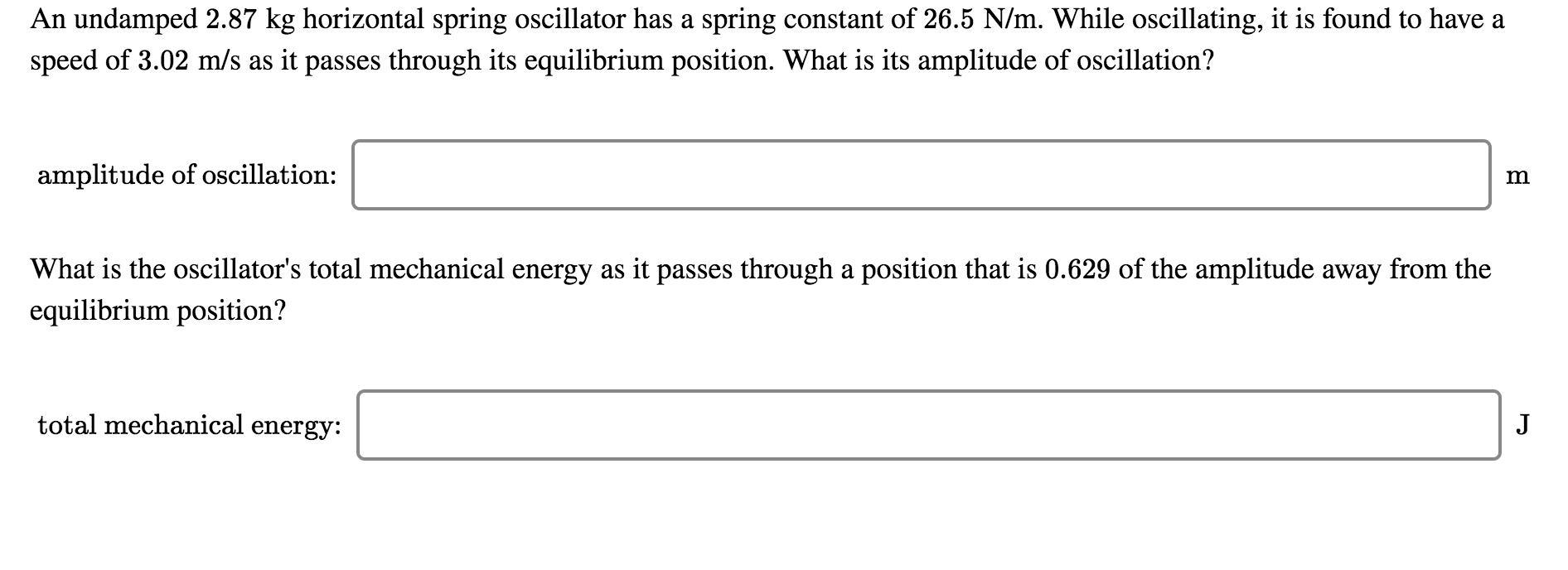An undamped 2.87 kg horizontal spring oscillator has a spring constant of 26.5 N/m. While oscillating, it is found to have a speed of 3.02 m/s as it passes through its equilibrium position. What is its amplitude of oscillation? amplitude of oscillation: What is the oscillator's total mechanical energy as it passes through a position that is 0.629 of the amplitude away from the equilibrium position? total mechanical energy:

• ### An undamped 2.36-kg horizontal spring oscillator has a spring constant of 24.0 N/m. While oscillating, it...

An undamped 2.36-kg horizontal spring oscillator has a spring constant of 24.0 N/m. While oscillating, it is found to have a speed of 2.70 m/s as it passes through its equilibrium position. What is its amplitude of oscillation? 0.846m What is the oscillator\'s total mechanical energy as it passes through a position that is 0.794 of the amplitude away from the equilibrium position? _______J

• ### An undamped 1.97 kg horizontal spring oscillator has a spring constant of 38.8 N/m. While oscillating,...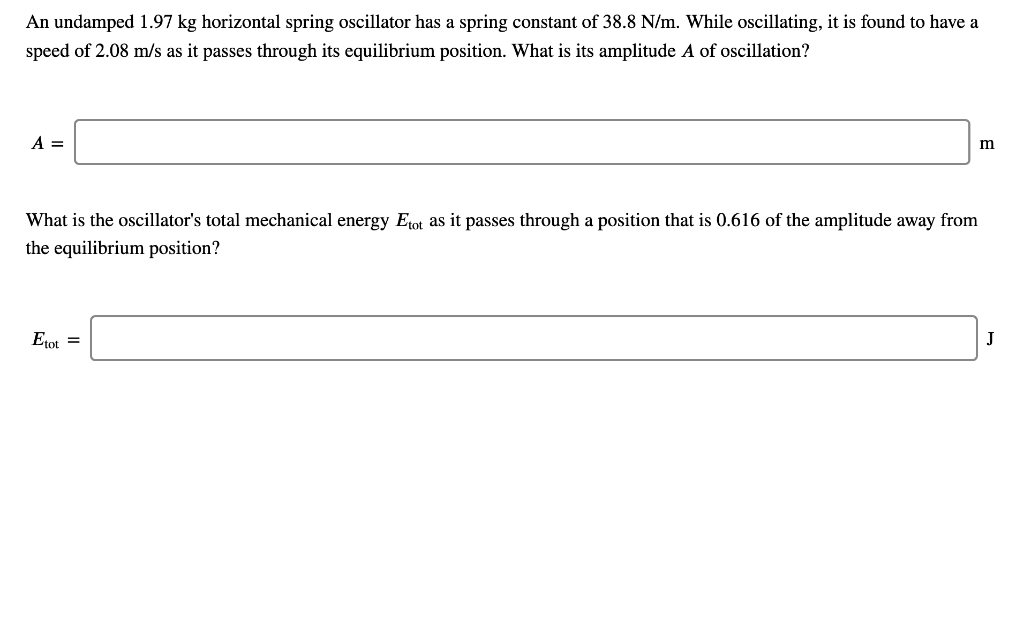An undamped 1.97 kg horizontal spring oscillator has a spring constant of 38.8 N/m. While oscillating, it is found to have a speed of 2.08 m/s as it passes through its equilibrium position. What is its amplitude A of oscillation? A = m What is the oscillator's total mechanical energy Etot as it passes through a position that is 0.616 of the amplitude away from the equilibrium position? Etot = J

• ### 2. (25 points) A 50.0 g ball stretches a spring by 2.45 cm when hanged vertically....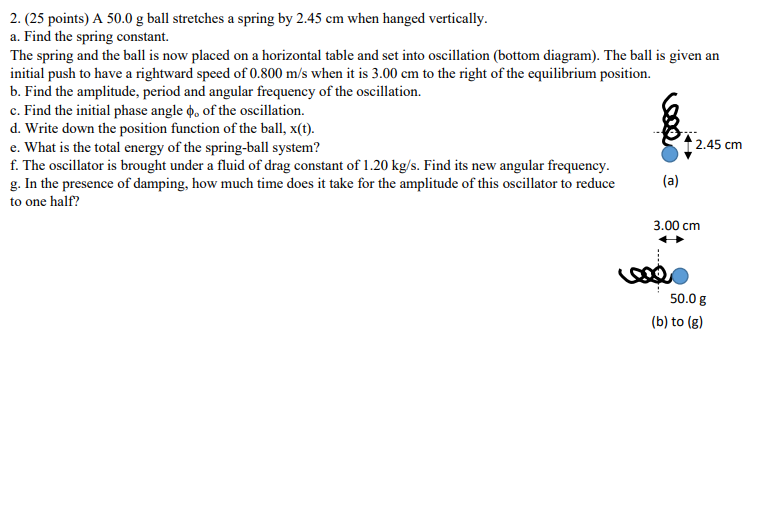2. (25 points) A 50.0 g ball stretches a spring by 2.45 cm when hanged vertically. a. Find the spring constant. The spring and the ball is now placed on a horizontal table and set into oscillation (bottom diagram). The ball is given an initial push to have a rightward speed of 0.800 m/s when it is 3.00 cm to the right of the equilibrium position. b. Find the amplitude, period and angular frequency of the oscillation. c. Find the...

Free Homework Help App